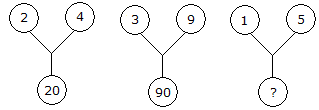# Verbal Reasoning - Character Puzzles - Discussion

### Discussion :: Character Puzzles - Character Puzzles 1 (Q.No.21)

21.

Which one will replace the question mark ?[A]. 75 [B]. 26 [C]. 25 [D]. 20

Explanation:

(2)2 + (4)2 = 20

and (3)2 + (9)2 = 90

Therefore (1)2 + (5)2 = 26.

 Darkprince said: (Sep 3, 2011) One more also possible :- 2 ^ 4 + 4 = 20 3 ^ 4 + 9 = 90

 Nivya said: (Nov 9, 2020) Super, thank you @Darkprince.

 Shubham said: (Mar 4, 2021) According to me, 2*4 = 8, 8*2 + 4 = 20. 3*9 = 27, 27*3 + 9 = 90.

 Nabukalu Aidah said: (May 8, 2021) Thanks for explaining.